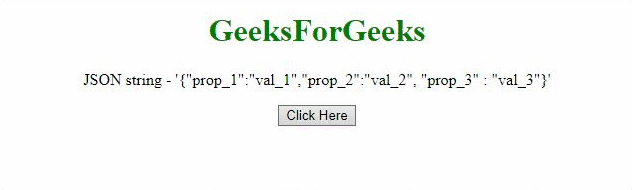# How to convert JSON string to array of JSON objects using JavaScript ?

Given a JSON string and the task is to convert the JSON string to an array of JSON objects. This array contains the values of JavaScript objects obtained from the JSON string with the help of JavaScript. There are two approaches to solve this problem which are discussed below:

Approach 1: First convert the JSON string to the JavaScript object using JSON.Parse() method and then take out the values of the object and push them into the array using push() method.

Example:

## html

 `` `<``html``>`   `<``head``>` `    ``<``title``>` `        ``How to convert JSON string to array` `        ``of JSON objects using JavaScript?` `    ``` ``   `<``body` `style` `= ``"text-align:center;"``>` `    `  `    ``<``h1` `style` `= ``"color:green;"` `>` `        ``GeeksForGeeks` `    ``` `    `  `    ``<``p` `id` `= ``"GFG_UP"``>` `    `  `    ``<``button` `onclick` `= ``"myGFG()"``>` `        ``Click Here` `    ``` `    `  `    ``<``p` `id` `= ``"GFG_DOWN"``>` `    `  `    ``<``script``>` `        ``var up = document.getElementById("GFG_UP");` `        `  `        ``var JS_Obj =` `        ``'{"prop_1":"val_1", "prop_2":"val_2", "prop_3" : "val_3"}';` `        `  `        ``up.innerHTML = "JSON string - '" + JS_Obj + "'";` `        `  `        ``var down = document.getElementById("GFG_DOWN");` `        `  `        ``function myGFG() {` `            ``var obj = JSON.parse(JS_Obj);` `            ``var res = [];` `            `  `            ``for(var i in obj)` `                ``res.push(obj[i]);` `            `  `            ``down.innerHTML = "Array of values - ["` `                            ``+ res + "]";` `        ``}` `    ``` ``   ``

Output:Approach 2: This approach is also the same but uses a different method. Convert the JSON string to the JavaScript object using eval() method and then take out the values of the object and push them to the array using push() method.

Example:

## html

 `` `<``html``>`   `<``head``>` `    ``<``title``>` `        ``How to convert JSON string to array` `        ``of JSON objects using JavaScript?` `    ``` ``   `<``body` `style` `= ``"text-align:center;"``>` `    `  `    ``<``h1` `style` `= ``"color:green;"` `>` `        ``GeeksForGeeks` `    ``` `    `  `    ``<``p` `id` `= ``"GFG_UP"``>` `    `  `    ``<``button` `onclick` `= ``"myGFG()"``>` `        ``Click Here` `    ``` `    `  `    ``<``p` `id` `= ``"GFG_DOWN"``>` `    `  `    ``<``script``>` `        ``var up = document.getElementById("GFG_UP");` `        `  `        ``var JS_Obj =` `        ``'{"prop_1":"val_1", "prop_2":"val_2", "prop_3" : "val_3"}';` `        `  `        ``up.innerHTML = "JSON string - '" + JS_Obj + "'";` `        `  `        ``var down = document.getElementById("GFG_DOWN");` `        `  `        ``function myGFG() {` `            ``var obj = eval('(' + JS_Obj + ')');` `            ``var res = [];` `            `  `            ``for(var i in obj)` `                ``res.push(obj[i]);` `            `  `            ``down.innerHTML = "Array of values - ["` `                            ``+ res + "]";` `        ``}` `    ``` ``   ``

Output:JavaScript is best known for web page development but it is also used in a variety of non-browser environments. You can learn JavaScript from the ground up by following this JavaScript Tutorial and JavaScript Examples.

Whether you're preparing for your first job interview or aiming to upskill in this ever-evolving tech landscape, GeeksforGeeks Courses are your key to success. We provide top-quality content at affordable prices, all geared towards accelerating your growth in a time-bound manner. Join the millions we've already empowered, and we're here to do the same for you. Don't miss out - check it out now!

Previous
Next